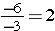# Integer OperationsIn the previous lesson, you learned to use the number line to show the overall change involving negative and positive integers. You also had a chance to review absolute value and how it can be used to determine distance above or below 0.

In this lesson, you will focus on mathematical operations involving negative and positive integers.

For example, you could be playing a game where if you answer the question correctly, you would receive 5 points, and if you answer incorrectly, you might lose 5 points. Remember that the numbers 5 and -5 are opposites because they are both the same distance from zero on the number line and have the same absolute value. Now, let us add your points together using this idea. If I gain 5 points, and then lose 5 points, it is like moving to the right 5 spaces from 0 and moving back to the left 5 spaces. Therefore, my score is 0. Two numbers that have a sum of zero are additive inverses.

If the signs are the same, combine by adding the absolute value of each number and keep the same sign. If the signs are different, ignore them. Then subtract the small number from the larger number and keep the sign of the original number that has the larger absolute value.

### Example One

-5 + (-3)     same signs

|-5| + |-3| = 5 + 3 = 8    add the absolute value of each number

-5 + (-3) = – 8     keep the original sign

### Example Two

Add – 5 + 3     opposite signs

|-5| – |3| = 5 – 3 = 2     subtract the smaller number from the larger number

5 is greater than 3, so -5 + 3 = -2     keep the sign of the original number that has the larger absolute value

One easy way to remember this rule is:

SSS Same Sign Sum (When the signs are alike, find the sum (add) and use the given sign)

DSD Different Signs Difference (When signs are different, find the difference (subtract) and use the sign of the larger absolute value.)

### Investigate

1. A fisherman drops his net to a depth of 12 feet below the surface of the water. How far does he need to raise the net to bring it back to the surface of the water?
2. A bird is 22 feet above the ground. What integer would you use to represent the distance that the bird needs to fly to get back to the ground?

What happens when you subtract positive and negative numbers? Because addition and subtraction are actually inverse operations, you can rewrite subtraction expressions as addition expressions.

Think about a simple problem like this one: 6 – 4 = ______

Rewrite it as an addition problem: 6 + (-4) = _______

Both expressions will simplify to positive 2, so you can see that subtraction can be written as addition, just by following one simple rule: (Add the opposite)

From Example One above: -5 + (-3)  same as – 5 – 3

## Subtraction Rule

Adding the opposite of the second number gives the same answer as subtracting that number.

First: Change the subtraction sign to an addition sign

Second: Change the sign of the integer you are subtracting

Third: Use the integer addition rules

### Example

6 – (-3) = 6 + (+3) = 9

One easy way to remember this rule:

KCC (Keep, Change, Change) (Keep the first number, change subtraction to addition, change second number)

### Investigate

1. Bo is playing a game online. If he gets 25 points, he will have a new high game. He currently has 5 points. What is the difference between the number of points he needs for a new high game and the number of points he currently has?
2. The temperature at noon is -7°F. The temperature at 6:00 PM is – 18°F. What is the difference in the temperatures?

### Multiplication and Division

There is set of rules to learn as you solve operations with integers and it applies to both integer multiplication and division.

Multiplication Rule: When the signs are alike, the product is positive and when the signs are different, the product will be negative.

### Example

1. (2)(3)   =  6
2. (-2)(-3) =  6
3. (-2)(3)  = -6
4. (2)(-3)  = -6

### Investigate

• A movie rental company deducts \$9 from Dulce’s account every month for a year. After 1 year, how much do movie rental fees change Dulce’s account?

Division Rule: The rules for determining the sign of the answer for division of integers are the same for multiplication. When the signs are alike, the answer is positive and when the signs are different, the answer will be negative.

### Example

1.   6  ÷  3   =  2
2.  -6  ÷ -3  =   2
3.  -6  ÷  3  =  -2
4.   6  ÷ -3  =  -2

These problems may also be written as### Investigate

• Cameron rode his bicycle 350 feet down to the bottom of a hill. He completed this ride in 5 equal stages. How much did Cameron’s elevation change during each stage of the ride?

## Practice

1)  -4 + -2

2)  7 + (-9)

3)  31 + (-20)

4)  -5 + (-7)

5)  41 – 15

6)  59 + (-59)

7)  59 – (-59)

8)  (-2) * (-2)

9)  (7) * (-40)

10)  (-7) * (-40)

(source)